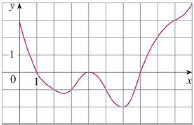Chapter 5.2, Problem 5E### Single Variable Calculus: Early Tr...

8th Edition
James Stewart
ISBN: 9781305270343

#### Solutions

Chapter
Section### Single Variable Calculus: Early Tr...

8th Edition
James Stewart
ISBN: 9781305270343
Textbook Problem

# The graph of a function f is given. Estimate ∫ 0 10 f ( x ) d x using five subintervals with (a) right endpoints, (b) left endpoints, and (c) midpoints.(a)

To determine

To estimate: The value of the integral 010f(x)dx using right end points.

Explanation

Given information:

The graph of the function 010f(x)dx.

The integral function is 010f(x)dx.

Calculation:

The expression Riemann sum using right end points is shown below:

Rn=i=1nf(xi)Δx=Δx(f(x1)+.......+f(xn)) (1)

Apply the condition for Riemann sum as follows:

Δx=ban (2)

Here, width of the subinterval is Δx, the upper limit is b, the lower limit is a, the number of subinterval is n, and the right endpoint of (xi1,xi) is xi.

Find the width of the subinterval (Δx) using Equation (2) as shown below.

Consider the number of subintervals as 5.

Substitute 5 for n, 10 for b and 0 for a in Equation (2).

Δx=1005=2

Calculate the value of Riemann sum using right end points

(b):

To determine

To estimate: The value of the integral 010f(x)dx using left end points.

(c)

To determine

To estimate: The value of the integral 010f(x)dx using midpoints.

### Still sussing out bartleby?

Check out a sample textbook solution.

See a sample solution

#### The Solution to Your Study Problems

Bartleby provides explanations to thousands of textbook problems written by our experts, many with advanced degrees!

Get Started

#### Find more solutions based on key concepts